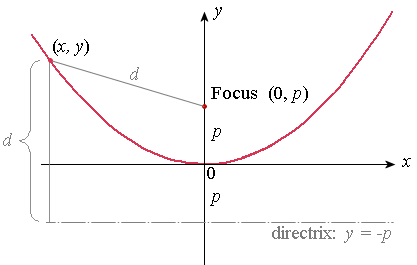## 抛物线图像计算器

–  o  +  ←  ↓  ↑  →
5
10
-5
-10
5
-5
x
y
D

Directrix

F
P
Q

d = 1.81

d = 1.81

### 主要工具

You can move the graph up-down, left-right if you hold down the "Shift" key and then drag the graph.

The parabola is defined as the locus of a point which moves so that it is always the same distance from a fixed point (called the focus) and a given line (called the directrix).

The focus of the parabola is at (0,p).

The directrix is the line y=−p.

The focal distance is |p| (Distance from the origin to the focus, and from the origin to the directrix. We take absolute value because distance is positive.)

The point (x, y) represents any point on the curve.

The distance d from any point (x, y) to the focus (0,p) is the same as the distance from (x, y) to the directrix.